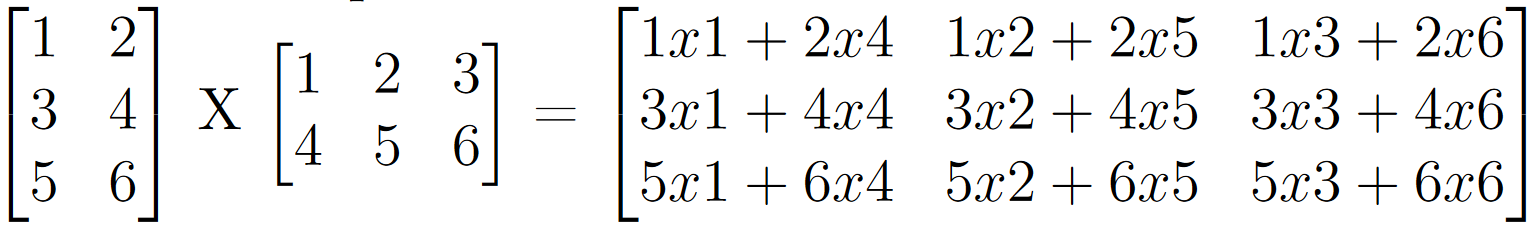Recent Tutorials and Articles
Optimizing Multiplication Operations in Matrices Multiplication
Published on: 17th January 2019

This tutorial provides an algorithm to optimize multiply operations while performing multiplication of many matrices.

# Problem Statement

We need to find optimized order of multiplication of N matrices in a way that no of multiply operations of matrix elements are minimum.

## Assumptions:

• Only adjacent matrices can be multiplied
• All adjacent matrices are multiplication compatible so no need to handle those conditions

## Example:

Suppose we need to multiply following four matrics with given dimensions -

• A - 2 X 2
• B - 2 X 3
• C - 3 X 4
• D - 4 X 2

Optimized order of multiplication will be - (A X B) X (C X D). Basically, if we first multply A by B and C by D and then multiply matrices from output of these, we will only have 44 multiplications of matrix elements which is minimum considering all possible ways of multiplying the given matrices.

# Formula for calculating Multiply Operations in Matrix Multiplication

Firstly, we will find out formula for calculating number of multiplication operations while multiplying two matrices.

For example, we have following matrices of dimensions 3 X 2 and 2 X 3.As shown above, we need 18 multiplications for multiplying these two matrices.

We can generalize this for multiplication of two matrices with dimensions N X M and M X P as -

No of Multiplication Operations = N X M X P  where N is no of rows in first matrix, P is no of columns in second matrix and M is no of columns in first matrix or rows in second matrix

# Algorithm for Optimizing Multiply operations in Matrices Multiplication

Here is the algorithm that we will employ to find order of multiplication of many matrices that optimizes number of multiply operations of their elements

1. Assign no of remaining matrices to no of matrices
2. Initialize final order of multiplication of matrices to empty string
3. Initialize total number of multiply operations to 0
4. Iterate over number of remaining matrices until it is greater than one
• Go through all the matrices and find adjacent matrices whose multiplication will result into highest multiply operations (see the formula from previous section) and yield smaller matrices (rows and cols)
• Add these two matrices to final order of multiplication of matrices
• Add no of multiply operations from these two matrices to total number of multiply operations
• Update first matrix dimensions with yield matrix and nullify second matrix
5. Print final order of matrices multiplication
6. Print total number of multiply operations needed while multiplying given matrices

# Java Implementation of Algorithm

``````package com.aksain.data.structures;

import java.util.Arrays;
import java.util.Scanner;

public class OptimizedMatricesMultiplication {
private static class MatrixDim {
int noOfRows;
int noOfCols;
public MatrixDim(String rowColStr) {
String[] rowCols = rowColStr.split(" ");
if(rowCols.length < 2) {
throw new IllegalArgumentException("Incorrect Matrix Dimensions. Expected - 'No_Of_Rows No_Of_Cols'");
}
this.noOfRows = Integer.parseInt(rowCols);
this.noOfCols = Integer.parseInt(rowCols);
}
}

public static void main(String[] args) {
// Read matrices information from console input
MatrixDim[] matrixDimensions;
try(final Scanner scanner = new Scanner(System.in)) {
System.out.println("Enter number of Matrices to be multiplied: ");
final int noOfMatrices = Integer.parseInt(scanner.nextLine());

matrixDimensions = new MatrixDim[noOfMatrices];
for(int i = 0; i < noOfMatrices; i++) {
System.out.println("Enter dimensions(<NoOfRows> <NoOfCols>) of Matrix " + i + ":");
matrixDimensions[i] = new MatrixDim(scanner.nextLine());
}
}

int remainingMatrices = matrixDimensions.length;
final StringBuilder finalMatrixMultiplyOrder =  new StringBuilder("");
int totalMultiplicationsNeeded = 0;
while(remainingMatrices > 1) {
// Idea is to multiply pair of matrices with more multiplications and yielding smaller matrix
int prevMatrixIndex = 0;
int maxMultiplicationsForCurrentIteration = 0;
int minMultipliedMatDim = Integer.MAX_VALUE;
String matrixIndexesForCurrentIteration = null;
for(int i = 1; i < matrixDimensions.length; i++) {
final MatrixDim prevMatrix = matrixDimensions[prevMatrixIndex];
if(matrixDimensions[i] == null) {
continue;
}
// find out dimensions of matrix from result of multiplication of previous and current ones
int multipliedMatrixDim = prevMatrix.noOfRows * matrixDimensions[i].noOfCols;
int noOfMultiplies = multipliedMatrixDim * prevMatrix.noOfCols;
// Reset max multiplications needed and matrix indexes for current iteration
if(maxMultiplicationsForCurrentIteration <= noOfMultiplies && minMultipliedMatDim > multipliedMatrixDim) {
maxMultiplicationsForCurrentIteration = noOfMultiplies;
matrixIndexesForCurrentIteration =  prevMatrixIndex + " " + i;
}

prevMatrixIndex = i;
}
// Add the indexes of finalized pair of matrices to final order
finalMatrixMultiplyOrder.append(matrixIndexesForCurrentIteration).append("\n");

// Multiply pair of matrices, update the first index with new rows & cols and make second index value null
final Integer[] matrixIndexes = Arrays.stream(matrixIndexesForCurrentIteration.toString().split(" ")).map(Integer::parseInt).toArray(Integer[]::new);
final MatrixDim leftMatrix = matrixDimensions[matrixIndexes];
final MatrixDim rightMatrix = matrixDimensions[matrixIndexes];
leftMatrix.noOfCols = rightMatrix.noOfCols;
matrixDimensions[matrixIndexes] = null;

totalMultiplicationsNeeded += maxMultiplicationsForCurrentIteration;

remainingMatrices--;
}
System.out.println("Final Matrices Multiplication Order: ");
System.out.println(finalMatrixMultiplyOrder);
System.out.println("Total Multiplications Needed: " + totalMultiplicationsNeeded);
}
}``````

## Sample Execution

``````Enter number of Matrices to be multiplied:
4
Enter dimensions(<NoOfRows> <NoOfCols>) of Matrix 0:
2 2
Enter dimensions(<NoOfRows> <NoOfCols>) of Matrix 1:
2 3
Enter dimensions(<NoOfRows> <NoOfCols>) of Matrix 2:
3 4
Enter dimensions(<NoOfRows> <NoOfCols>) of Matrix 3:
4 2
Final Matrices Multiplication Order:
2 3
1 2
0 1

Total Multiplications Needed: 44
``````

Thank you for reading through the tutorial. In case of any feedback/questions/concerns, you can communicate same to us through your comments and we shall get back to you as soon as possible.

Published on: 17th January 2019